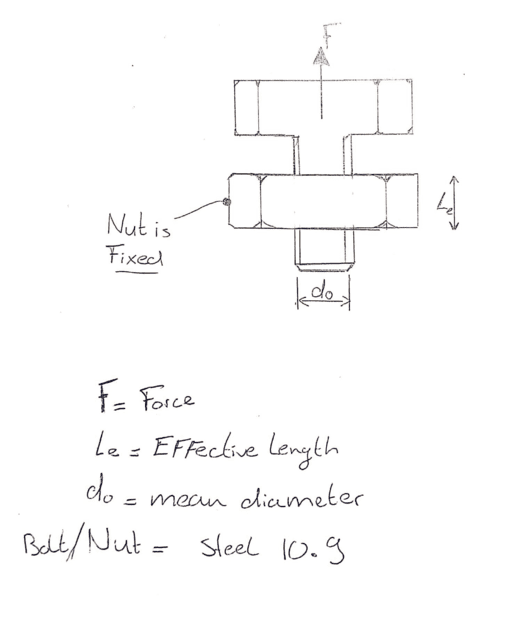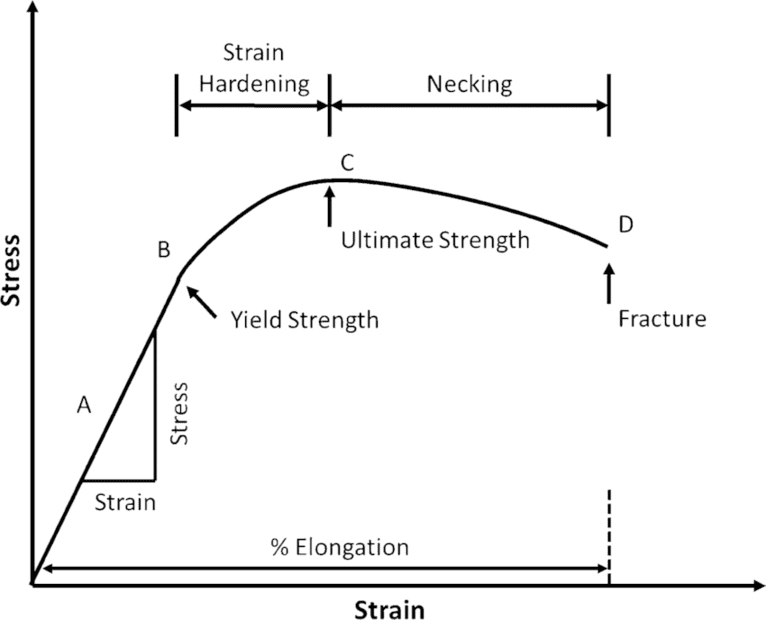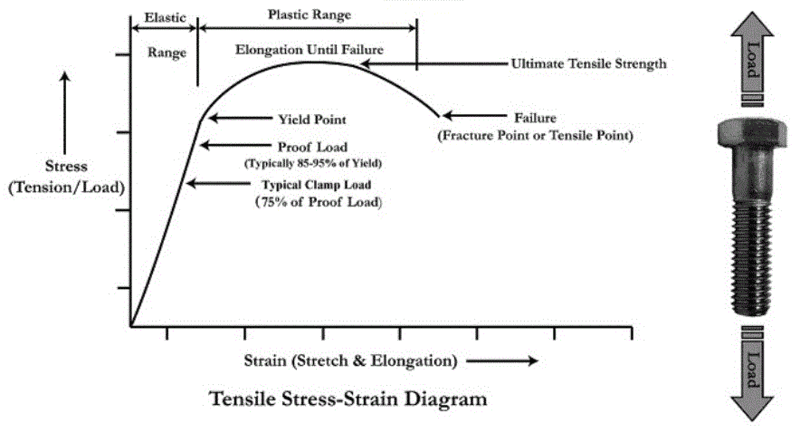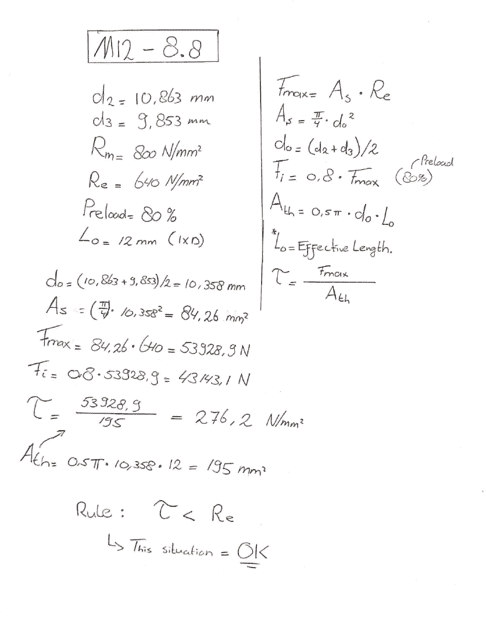# Determine the strength of a Bolt-Nut combination

Mech_LS24
TL;DR Summary
I want to determine by calculations how many force can be applied to a Bolt-Nut connection. Needed to now what input and formulas are necessary for this.
Hello!

From below sketch, I determined the following assumptions:
- Bolt/Nut: M12, Steel 10.9
- The nut is fixed

How can I calculate the ultimate force before the bolt will damage the nut? I can imagine the flanges of the threads will break. In documentation I have read about tension surface (At), Shear stress surface (Ath) and maximum shear stress (Τ). An explanation about these will be very helpfull!

Like to hearHomework Helper
Gold Member

•berkeman
Homework Helper
Gold Member
•Mech_LS24
Mech_LS24
From the documentation, I understand:

The strength of the thread is determined by the stress area. This stress area is the weakest point of the bolt.
Stress area = At = (phi/4)*d0^2 [mm^2]

The clamp load/preload is the same as the bolt strength. The bolt strength can be calculated with:
Fbolt = 0.75*(proof load)*(stress area) [N]

Questions:
• What is preload (Newton) different from tightening torque (Newton Meter)? This has interfaces with each other, right?
• Where does the 0.75 comes from in the formula of the bolt strength?
• The bolt strength is the maximum force till where the bolt breaks? Shown in the first video at 02:17
• The "proof load" is the peak of load (in Newton) where the bolt return to his original state? Like the yield strength?
Thanks

Mech_LS24
Thanks for your involvement @jrmichler , I appreciate the books but unfortunately I can't afford books of €150,-. Besides, I like this forum and being interactive with experienced persons.

As learing curve I prefer having starting points from the experienced people on where to search, that's why I am reach out here at this forum. After this starting point, I use the books as references. This is just the way I like it, maybe other persons have different approaches.

Mentor

I note that your questions in Post #4 are different from the questions in Post #1, which shows how the answer to one question leads to another, and another, and so on. Your curiosity is another reason to get a book. A book that covers many of your current and future questions is not an expense, but an investment.

Do you have access to an engineering library? Does the local library in your country have interlibrary loan capabilities?

Mech_LS24
I note that your questions in Post #4 are different from the questions in Post #1, which shows how the answer to one question leads to another, and another, and so on. Your curiosity is another reason to get a book. A book that covers many of your current and future questions is not an expense, but an investment.
I like reading books for sure. But often I do miss practical example in those books, it is explained in a general way and for a beginner in engineering it's hard to understand that. At least, that's how I feel it. When purchasing a book for that money and after reading a couple of chapters, getting the feeling I doesn't understand it and then avoid reading further the book is a frustrating thing. I totally agree a book is an investment.

Do you have access to an engineering library? Does the local library in your country have interlibrary loan capabilities?
The local library isn't supported with engineering books. I take notice of the engineering library, trying to find it here. Thanks for thatonatirec
Questions:
• What is preload (Newton) different from tightening torque (Newton Meter)? This has interfaces with each other, right?
You apply a torque to tighten bolts. This translates to an axial clamping force (or preload). There are equations relating the bolt torque to the resulting preload. It depends on a variety of factors that are difficult to estimate very accurately (thread and under-head frictions for example).

• Where does the 0.75 comes from in the formula of the bolt strength?
The clamp load/preload is the same as the bolt strength. The bolt strength can be calculated with:
Fbolt = 0.75*(proof load)*(stress area) [N]
The clamp/load is not the same as the bolt strength. Bolt proportional limit = proof strength = (proof load)*(stress area). The equation is above is calculating 75% of the bolt proportional yield limit. When specifying a preload, oftentimes a target is 60%-90% the proof strength of the bolt.
• The bolt strength is the maximum force till where the bolt breaks? Shown in the first video at 02:17
Bolts have yield strength, proof strength, and ultimate tensile strength; yield and proof are very similar and correlate to when plastic deformation starts. Generally speaking, exceeding the ultimate strength is when something actually breaks.
• The "proof load" is the peak of load (in Newton) where the bolt return to his original state? Like the yield strength?
Proportional limit is when the elasticity of the bolt begins to deviate from a linear Hookean relationship (stress = E*e). This occurs just before the actual elastic limit is reached. Loading a bolt to the proof strength and unloading would be purely elastic. However, the proportional limit is often measured and reported with an offset. You should look further into that to understand it better.

Shigleys is a great reference, but there are plenty of sources out there for free.

Roymech.org is one such free website that comes to mind.

EDIT: also, just like to add that older versions of textbooks can often be had for a few dollars. Oftentimes, the content is almost exactly the same and the exercise problems are changed; sometimes an extra section or two is added, but most of this fundamental information should still be available in older versions. I like the site bookfinder.com which scrapes data from several sites that sell books.

Last edited:
•Mech_LS24 and Lnewqban
Mech_LS24
You apply a torque to tighten bolts. This translates to an axial clamping force (or preload). There are equations relating the bolt torque to the resulting preload. It depends on a variety of factors that are difficult to estimate very accurately (thread and under-head frictions for example).
Hmm, so the preload is more or less the tightening torque needed to fasten a bolt?

The clamp/load is not the same as the bolt strength. Bolt proportional limit = proof strength = (proof load)*(stress area). The equation is above is calculating 75% of the bolt proportional yield limit. When specifying a preload, oftentimes a target is 60%-90% the proof strength of the bolt.
Shouldn't be proof strength = (proof load)/(stress area)? This results in N/mm^2 which is sigma (σ)? But this isn't the amount of force what can be applied to the bolt? The video shows that he applies an amount of force to the bolt/screw. By reaching his limit, the bolt breaks...

Bolts have yield strength, proof strength, and ultimate tensile strength; yield and proof are very similar and correlate to when plastic deformation starts. Generally speaking, exceeding the ultimate strength is when something actually breaks.
Is like a stress-strain curve? Whereby the proof strength is between yield strength and ultimate strength below?This gives me a good understanding!

However, the proportional limit is often measured and reported with an offset. You should look further into that to understand it better.
I am going to check that out :)

Shigleys is a great reference, but there are plenty of sources out there for free.

Roymech.org is one such free website that comes to mind.

EDIT: also, just like to add that older versions of textbooks can often be had for a few dollars. Oftentimes, the content is almost exactly the same and the exercise problems are changed; sometimes an extra section or two is added, but most of this fundamental information should still be available in older versions. I like the site bookfinder.com which scrapes data from several sites that sell books.
Thanks for mentioning! I have a Shigleys book now, but the USA standards are difficult haha. I need to adapt myself a bit.

onatirec
Shouldn't be proof strength = (proof load)/(stress area)?
Woops, yes, you are right.

These quantities are determined experimentally. I believe reported proof strength is often the minimum and not the mean.

Bolt installation can be specified by a tightening torque, resulting axial preload, or bolt elongation. At the end of the day, it's the axial preload or clamping force that keeps the joint together.

Is like a stress-strain curve? Whereby the proof strength is between yield strength and ultimate strength below?
Proof strength is slightly below yield, otherwise loading to proof and unloading would not be purely elastic. It correlates to the proportional limit.•Lnewqban and Mech_LS24
Homework Helper
Gold Member
From the documentation, I understand:

The strength of the thread is determined by the stress area. This stress area is the weakest point of the bolt.
Stress area = At = (phi/4)*d0^2 [mm^2]

The clamp load/preload is the same as the bolt strength. The bolt strength can be calculated with:
Fbolt = 0.75*(proof load)*(stress area) [N]
Unless you are designing very critical bolt-nuts assemblies, which failure could cause damage to property and persons, you could do your estimates following some rules of thumb.

If the thread area is small, it could fail due to shear of the threads by the axial force on the bolt (induced by applied torque, for clamping or other purposes).
For that reason, the threaded depth (for nuts or threaded holes of same materials) is recommended to be at least one diameter of the bolt, or 1.5 diameters for soft-hard combination of materials, such as steel and aluminum.

If that rule is followed, most likely the bolt shaft will fail due to axial force before the thread fails due to shear force.
Hope roughly responds your initial question:
"How can I calculate the ultimate force before the bolt will damage the nut? I can imagine the flanges of the threads will break."

The clamp force is whatever force the combination of torque and thread pitch creates in the direction axial to the bolt.
Yes, it could reach a maximum value just before the bolt snaps.
The bolt could be subjected to a combined load of axial, shear (perpendicular direction to the axis of the bolt) and even bending, for many actual situations.
The combination of those loads, sometimes accidental, could overwhelm the resistance of a bolt, which has been inducing a reduced clamping force.

Last edited:
•Mech_LS24
Mech_LS24
Thanks @Lnewqban .

I think I do understand it (in slow steps) a bit better now. I did the following calculation:

Conclusion of this calculation is a bolt (M12 in this case) with an effective length of 12 mm (threaded length in counterpart), is able to handle Fmax forces of 53928 N. As well I found that if an effective length of 4 mm is chosen, T will be higher as the Re so this will make the nut (or a threaded hole) break.

Correct? :)•Lnewqban